Home· Order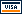· Contact us· AboutShareware· PMVR· SlideShowJava Tools· JexePack· Jobfuscate· MakeInstallAffordware· PhotoFinder· PrintEnvelope· WinOpenLearning + Fun· NX 101· Pano Help· Bug Free C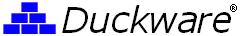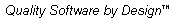You are here: Duckware » Technology » World's Hardest Easy Geometry Problem Contact us
World's Hardest Easy Geometry Problem

 Another puzzle: Can you solve this frog riddle (a math probability problem)?

Here are the World's Hardest Easy Geometry Problem, as found on Keith Enevoldsen's Think Zone web page: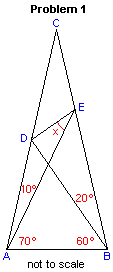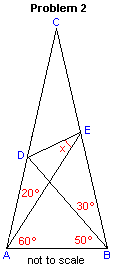Solutions to both of these problems can be found far below -- so be careful how far you scroll below if you don't want to see the answer.

What makes these geometry problems so interesting (and 'hard') is that only elementary geometry is allowed (no trigonometry). Like, basic rules about parallel and intersecting lines and the angles formed: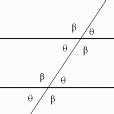as well as simple triangle facts:
• the sum of interior angles of a triangle add up to 180°
• isosceles triangle theorem about two equal angles/sides
• an equilateral triangle has three 60° angles and three equal length sides
as well as what makes two triangles congruent: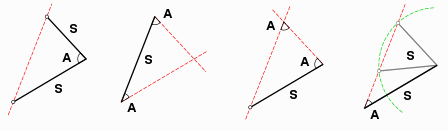The solutions below are by design not rigorous proofs, but rather provide just enough information to make the true solution very obvious to most readers.

You must click to find the images associated with these solutions -- so as to not spoil the fun for those that want to try to find the solution for themselves first.

Problem One Solution

Solution One Image

1. Calculate some known angles:
•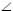ACB = 180-(10+70)-(60+20) = 20°
•AEB = 180-70-(60+20) = 30°
2. Draw a line from point D parallel to AB, labeling the intersection with BC as a new point F and conclude:
•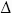DCFACB
•CFD =CBA = 60+20 = 80°
•DFB = 180-80 = 100°
•CDF =CAB = 70+10 = 80°
•ADF = 180-80 = 100°
•BDF = 180-100-20 = 60°
3. Draw a line FA labeling the intersection with DB as a new point G and conclude:
•ADF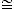BFD
•AFD =BDF = 60°
•DGF = 180-60-60 = 60° =AGB
•GAB = 180-60-60 = 60°
•DFG (with all angles 60°) is equilateral
•AGB (with all angles 60°) is equilateral
4.CFA with two 20° angles is isosceles, so FC = FA

5. Draw a line CG, which bisectsACB and conclude:
•ACGCAE
• FC-CE = FA-AG = FE = FG
• FG = FD, so FE = FD
6. With two equal sides,DFE is isosceles and conclude:
•DEF = 30+x = (180-80)/2 = 50
Answer: x = 20°

Problem Two Solution

Solution Two Image

1. Calculate some known angles:
•ACB = 180-(10+70)-(60+20) = 20°
•AEB = 180-60-(50+30) = 40°
2. Draw a line from point E parallel to AB, labeling the intersection with AC as a new point F and conclude:
•FCEACB
•CEF =CBA = 50+30 = 80°
•FEB = 180-80 = 100°
•AEF = 100-40 = 60°
•CFE =CAB = 60+20 = 80°
•EFA = 180-80 = 100°
3. Draw a line FB labeling the intersection with AE as a new point G and conclude:
•AFEBEF
•AFB =BEA = 40°
•BFE =AEF = 60°
•FGE = 180-60-60 = 60° =AGB.
•ABG = 180-60-60 = 60°
4. Draw a line DG. Since AD=AB (leg of isosceles) and AG=AB (leg of equilateral), conclude:
• AD = AG.
•DAG is isosceles
•ADG =AGD = (180-20)/2 = 80°
5. SinceDGF = 180-80-60 = 40°, conclude:
•FDG (with two 40° angles) is isosceles, so DF = DG
6. With EF = EG (legs of equilateral) and DE = DE (same line segment) conclude:
•DEFDEG by side-side-side rule
•DEF =DEG = x
•FEG = 60 = x+x
Answer: x = 30°